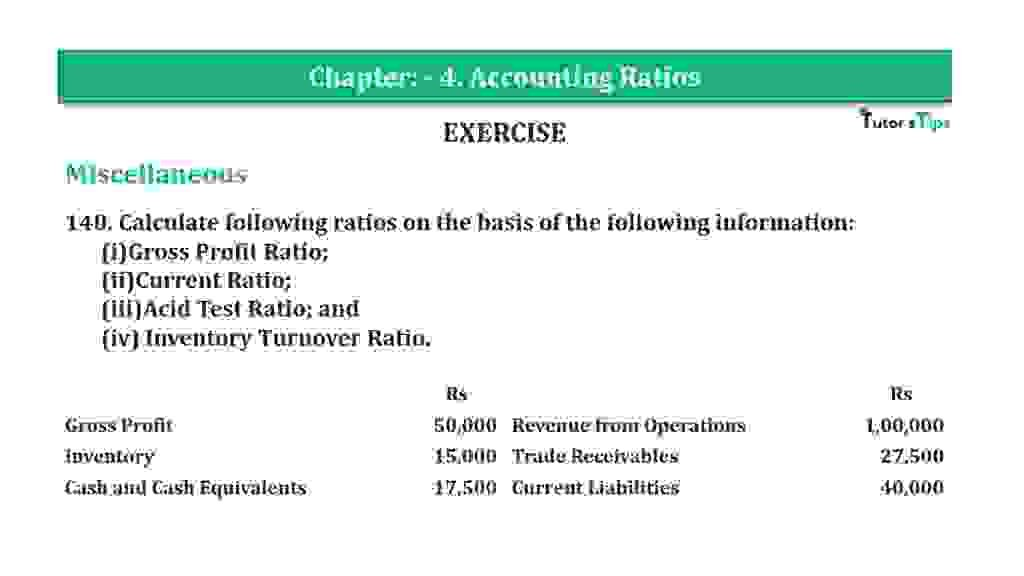# Question 140 Chapter 4 of +2-B – T.S. Grewal 12 ClassQuestion 140 Chapter 4 of +2-B

Miscellaneous

140. Calculate following ratios on the basis of the following information:
(i)Gross Profit Ratio;
(ii)Current Ratio;
(iii)Acid Test Ratio; and
(iv) Inventory Turnover Ratio.

 Rs Rs Gross Profit 50,000 Revenue from Operations 1,00,000 Inventory 15,000 Trade Receivables 27,500 Cash and Cash Equivalents 17,500 Current Liabilities 40,000

### The solution of Question 140 Chapter 4 of +2-B: –

I

 Gross Profit Ratio = Gross Profit X 100 Revenue from Operations
 Gross Profit Ratio = Rs. 50,000 X 100 Rs 1,00,000 = 50%

II

 Current Ratio = Current Assets Current Liabilities
 Current Assets = Inventory + Cash and Cash Equivalents + Trade Receivables = Rs 60,000 Current Liabilities = Rs 40,000

 Current Ratio = Rs. 60,000 Rs 40,000 = 1.5 : 1

III

 Liquid Ratio = Liquid Assets Current Liabilities
 Liquid Assets = Cash and Cash Equivalents + Trade Receivables = Rs 17,500 + Rs 27,500 = Rs 45,000
 = Rs 45,000 Rs 40,000 = 1.125 : 1

IV

 Inventory Turnover Ratio = Cost of Goods Sold Average Inventory
 Cost of Goods Sold = Revenue from Operations − Gross Profit = Rs 1,00,000 − Rs 50,000 = Rs 50,000 Average Inventory = Rs 15,000
 = Rs 50,000 Rs 15,000 = 3.33 Times

Balance Sheet: Meaning, Format & Examples

Thanks, Please Like and share with your friends

Comment if you have any question.

Also, Check out the solved question of previous Chapters: –

### T.S. Grewal’s Double Entry Book Keeping (Vol. II: Accounting for Companies)T.S. Grewal’s Analysis of Financial Statements